# FFT from a Vector (const Complex from a QVector)

• Hi guys,

I'm using this code do apply a FFT:

//------ Include FFT----------

``````#include <complex>
#include <iostream>
#include <valarray>
#include <algorithm>
``````
``````//------ Variables FFT----------

const double PI = 3.141592653589793238460;

typedef std::complex<double> Complex;
typedef std::valarray<Complex> CArray;
``````
``````//-------- FFT function--------

void fft(CArray& x)
{
const size_t N = x.size();
if (N <= 1) return;

// divide
CArray even = x[std::slice(0, N/2, 2)];
CArray  odd = x[std::slice(1, N/2, 2)];

// conquer
fft(even);
fft(odd);

// combine
for (size_t k = 0; k < N/2; ++k)
{
Complex t = std::polar(1.0, -2 * PI * k / N) * odd[k];
x[k    ] = even[k] + t;
x[k+N/2] = even[k] - t;
}
}
``````
``````//---------- Main function---------

void MainWindow::FFF_Plot(){

QVector<double> x, y;
QVector<double> x, y;
``````

//1 kHz sine wave (256 points)

``````    const Complex test[] = { 0, 0.247404, 0.479426, 0.681639, 0.841471, 0.948985, 0.997495, 0.983986, 0.909297, 0.778073, 0.598472,
0.381661, 0.14112, -0.108195, -0.350783, -0.571561, -0.756802, -0.894989, -0.97753, -0.999293, -0.958924,
-0.858934, -0.70554, -0.508279, -0.279415, -0.0331792, 0.21512, 0.450044, 0.656987, 0.823081, 0.938, 0.994599,
0.989358, 0.922604, 0.798487, 0.624724, 0.412118, 0.173889, -0.0751511, -0.319519, -0.544021, -0.734698, -0.879696,
-0.969998, -0.99999, -0.967808, -0.875452, -0.728665, -0.536573, -0.311119, -0.0663219, 0.182599, 0.420167, 0.631611,
0.803784, 0.925982, 0.990607, 0.993641, 0.934895, 0.818022, 0.650288, 0.442122, 0.206467, -0.0420244, -0.287903,
-0.515882, -0.711785, -0.863433, -0.961397, -0.999586, -0.975626, -0.891006, -0.750987, -0.564276, -0.342481, -0.0993915,
0.149877, 0.389827, 0.60554, 0.783603, 0.912945, 0.985525, 0.99683, 0.946156, 0.836656, 0.675136, 0.471639, 0.238818,
-0.00885131, -0.25597, -0.487175, -0.688088, -0.84622, -0.951738, -0.998082, -0.98237, -0.905578, -0.772483, -0.591358,
-0.373465, -0.132352, 0.11699, 0.359058, 0.578802, 0.762558, 0.898903, 0.979358, 0.998921, 0.956376, 0.854368, 0.69924,
0.500636, 0.270906, 0.0243315, -0.223756, -0.457931, -0.663634, -0.828076, -0.941031, -0.995479, -0.988032, -0.919154,
-0.793127, -0.617788, -0.404038, -0.165166, 0.0839745, 0.327894, 0.551427, 0.740674, 0.88387, 0.972112, 0.999912, 0.965542,
0.87114, 0.722574, 0.529083, 0.302695, 0.0574875, -0.191294, -0.428183, -0.638449, -0.809019, -0.929288, -0.991779, -0.992606,
-0.931717, -0.812899, -0.643538, -0.434166, -0.197799, 0.0508662, 0.296369, 0.523444, 0.717975, 0.867865, 0.963795, 0.999802,
0.973645, 0.886953, 0.745113, 0.556946, 0.334151, 0.0905802, -0.158623, -0.397963, -0.61256, -0.789071, -0.916522, -0.986987,
-0.996087, -0.943254, -0.831775, -0.66858, -0.463816, -0.230214, 0.0177019, 0.264517, 0.494885, 0.694484, 0.850904, 0.954418,
0.998591, 0.980676, 0.901788, 0.766831, 0.584197, 0.365239, 0.123573, -0.125776, -0.367305, -0.585997, -0.768255, -0.902746,
-0.981108, -0.998471, -0.953753, -0.849735, -0.692885, -0.492955, -0.262375, -0.0154818, 0.232374, 0.465781, 0.670229, 0.833005,
0.943989, 0.99628, 0.986628, 0.915631, 0.787705, 0.610804, 0.395925, 0.15643, -0.0927912, -0.336243, -0.558789, -0.746592,
-0.887976, -0.974149, -0.999755, -0.963201, -0.86676, -0.716427, -0.521551, -0.294247, -0.0486486, 0.199975, 0.436165, 0.645236,
0.81419, 0.932521, 0.992873, 0.991492, 0.928466, 0.807712, 0.636738, 0.426175, 0.189115, -0.0597041, -0.304811, -0.530966,
-0.724108, -0.872228, -0.966118, -0.999939, -0.971589, -0.88283, -0.739181, -0.549573, -0.325796, -0.0817617, 0.167356, 0.406068,
0.619532, 0.794478 };

CArray data(test, 256);

// forward fft
fft(data);

//   std::vector<double> real ;
for (int i = 0; i < 256; ++i)
{
y.append(data[i].real());
}

for (int i = 0; i < 256; ++i)
{
x.append(i*98.0392);
}

qDebug () << "X: "<< x;
qDebug () << "Y: "<< y;

}
``````

This code is working. But now I need to change the Array using points from a chart. The points are stored in a QVector. Therefore, QVector values ​​need to take the place of const Complex values. It's possible? What I should have to do?

Cheers

• @pedromenezes

How does the data looks like in your QVector?

I would suggest to iterate through the QVector and convert the data to a complex number. If the data are real numbers, the imaginary part is 0.

By the way, I'd recommend using FFT libraries, like FFTW. I use the Kiss FFT, which is really easy to use and quite fast. There you have already a optimized FFT for real data.

• Thank you very much @beecksche !!! I followed your advise and changed for Kiss FFT. I think that everything is working now. My only problem at this moment is my own limitation in c++.

So I did three function (ConvertRealtoComplex, ConvertComplextoReal and FFT) Could you help me? I need to convert a double to complex (I really did) and apply the FFT function.

Here are my functions:

``````
// 1. CONVERT REAL SIGNAL TO COMPLEX

void MainWindow::convertRealtoComplex(){

nfft=256; // number of samples (I'm using 256)
is_inverse_fft = 0;  // direct or inverse FFT (I'm using 0 to direct)

kiss_fftr_cfg cfg = kiss_fftr_alloc(nfft, is_inverse_fft, nullptr, nullptr);
kiss_fft_scalar *cx_in = new kiss_fft_scalar[nfft];
kiss_fft_cpx *cx_out = new kiss_fft_cpx[nfft/2+1];

// my `nfft` samples in cx_in[i]
//getting data from a plot

for(int i=0; i<nfft;i++){

cx_in[i] = ui->customPlot->graph(0)->data()->at(i)->value;

}

kiss_fftr(cfg, cx_in, cx_out);

// the complex number seems to be ok!

for(int i=0; i<nfft;i++){
qDebug () << "cx_in:["<< i << "]"<< cx_in[i];
qDebug () << "cx_out:[" << i << "]"<< cx_out[i].r << cx_out[i].i;
}

// Now I need to apply the FFT here using my function (FFT) and process the spectrum `cx_out` here: We have `nfft/2+1` (!) samples. But I don't know how. :(

free(cfg);
delete[] cx_in;
delete[] cx_out;
}

// 2. CONVERT COMPLEX TO REAL SIGNAL

void MainWindow::convertComplextoREAL(){

nfft=256;
is_inverse_fft = 1;

kiss_fftr_cfg cfg = kiss_fftr_alloc(nfft, is_inverse_fft, nullptr, nullptr);
kiss_fft_cpx *cx_in = new kiss_fft_cpx[nfft/2+1];
kiss_fft_scalar *cx_out = new kiss_fft_scalar[nfft];

// put kth frequency sample in cx_in[k] up to index `nfft/2`.
// No need to populate the mirror.

kiss_fftri(cfg, cx_in, cx_out);

// Process signal `cx_out` here. It has again `nfft` samples
// and is real valued.

free(cfg);
delete[] cx_in;
delete[] cx_out;
}

// 3. FFT FUNCTION
void MainWindow::FFT(){

nfft=256;
is_inverse_fft = 0;

kiss_fft_cfg cfg = kiss_fft_alloc( nfft, is_inverse_fft, nullptr, nullptr );
kiss_fft_cpx *cx_in = new kiss_fft_cpx[nfft];
kiss_fft_cpx *cx_out = new kiss_fft_cpx[nfft];

// put kth sample in cx_in[k].r and cx_in[k].i

kiss_fft(cfg, cx_in, cx_out);

// transformed. DC is in cx_out.r and cx_out.i

free(cfg);
delete[] cx_in;
delete[] cx_out;
}
``````

Cheers

• I need to convert a double to complex (I really did) and apply the FFT function.

I don't follow, what is the problem exactly? I haven't worked with that library, but I'm quite sure `kiss_fftr(cfg, cx_in, cx_out);` already applies the FFT to the dataset.

• @pedromenezes

The configurations of your FFT looks good, but like @kshegunov already mentioned: by calling `kiss_fftr` or `kiss_fftri` your perform a FFT of your data.

These functions `kiss_fftr` or `kiss_fftri` are optimized functions for real to complex and complex to real data.

Your function `MainWindow::FFT()` performs a FFT of complex to complex data.

So if you know your data are real and you want a frequency analysis (eg. analysis of a sampled signal) you can use the `kiss_fftr` function. And if you know that the complex data you have are values of a real function/data you can the `kiss_fftri` function. In all other cases, where you don't know what kind of data you have you should perform a complex to complex FFT (In worst case you get the conjugate values).

Below an example which may help you of real to complex and complex to real data:

``````void FFT()
{
QVector<kiss_fft_scalar> sampledSignal(256);
for (int i = 0; i < sampledSignal.size(); i++)
sampledSignal[i] = qCos(2.0*M_PI*i/256.0);

bool inverserFFT = false;
int nfft = sampledSignal.size();

QVector<kiss_fft_cpx> frequencies(nfft/2 + 1);

kiss_fftr_cfg cfg = kiss_fftr_alloc(nfft, inverserFFT, nullptr, nullptr);
kiss_fftr(cfg, sampledSignal.data(), frequencies.data());

free(cfg);

// now we do a inverse FFT of the complex to real data with kiss_fftri
// and save it in a new QVector sampledSignalFFT

QVector<kiss_fft_scalar> sampledSignalFFT(nfft);

inverserFFT = true;
kiss_fftr_cfg cfg_inverse = kiss_fftr_alloc(nfft, inverserFFT, nullptr, nullptr);
kiss_fftri(cfg_inverse, frequencies.data(), sampledSignalFFT.data());

free(cfg_inverse);

for (int i = 0; i < sampledSignalFFT.size(); i++) {
double v1 = sampledSignal.at(i);
double v2 = sampledSignalFFT.at(i) / nfft;
// v1 == v2
}
}
``````

If you want to transform complex to complex data you can either use the `kiss_fft` function or `kfc_fft` or `kfc_ifft` for inverser FFT.

``````void FFTComplex()
{
QVector<kiss_fft_cpx> complexInput(256);
for (int i = 0; i < sampledSignal.size(); i++)
complexInput[i].r = qCos(2.0*M_PI*i/256.0);   // imaginary part is initialized with 0

QVector<kiss_fft_cpx> complexOutput(256);

kfc_fft(256, complexInput.data(), complexOutput.data());

QVector<kiss_fft_cpx> complexInputFFT(256);
kfc_ifft(256, complexOutput.data(), complexInputFFT.data());

for (int i = 0; i < sampledSignalFFT.size(); i++) {
kiss_fft_cpx v1 = complexInput.at(i);
kiss_fft_cpx v2 = complexInputFFT.at(i);
v2.r /= nfft;

// v1 == v2
}
}
``````

If you perform a inverse FFT the data is not automatically devided by the size of data, so you need to divide by yourself with `nfft` (if needed).

• Thank you very much @kshegunov and @beecksche !! Now the FFT is working. If I do not bother you too much, I have just one more question!

The wave amplitude in the frequency domain suffers some interference (aliasing ??), which does not maintain the amplitude constant with the frequency variation (even with the constant amplitude in the time domain). From 1kHz it starts to decrease until it reaches the smallest amplitude at 1035 Hz, then it increases again and reaches its maximum amplitude at 1070 Hz (cycles of decrease and increase every 35 Hz), then the standard changes to 35 Hz (increase ), 60 (decrease), 35, 60 ... so on.

Here is my code on spin change (frequency change):

``````void MainWindow::makePlotWave() {

QVector<double> x(256), y(256);

int amp = ui->spinBox_2->value(); // to get the amplitude
int freq = ui->spinBox->value(); // to get the frequency
int phase = ui->spinBox_3->value(); //to get the phases
double sine = freq*2.0*M_PI;

for (int i=0; i<256; ++i)
{
x[i] = i*0.0416; // 0.0416 to show 256 points in 10.608 msec
double time = x[i]/1000;//time in (msec)
y[i] = amp*qSin(sine*(time)+phase);// Y = Amp*Sin(Freq*2*Pi*time + phase)
}
// after the data are plotted
}
``````

Here is my FFT function:

``````void MainWindow::FFT()
{
QVector<kiss_fft_scalar> sampledSignal(256), x(256);

for(int i=0; i<sampledSignal.size();i++){

sampledSignal[i] = ui->customPlot->graph(0)->data()->at(i)->value; /*to get y data from the plot*/
}

bool inverserFFT = false;
int nfft = sampledSignal.size();
int i_freq = nfft/2+1;

QVector<kiss_fft_cpx> frequencies(i_freq);

kiss_fftr_cfg cfg = kiss_fftr_alloc(nfft, inverserFFT, nullptr, nullptr);
kiss_fftr(cfg, sampledSignal.data(), frequencies.data());

free(cfg);

QVector <double> x_freq(i_freq), y_freq(i_freq);

for(int i=0; i<i_freq;i++){

x_freq[i] = i*100; /*to show correct spectrum values. I found out by testing, I still did not understand why I had to multiply by 100*/

}

for(int i=0; i<i_freq;i++){

y_freq[i] = abs(frequencies[i].r)/100; /*take real values, convert real values in absolutes values and adjust the amplitude*/
}

qDebug () << "X freq: " << x_freq;
qDebug () << "Y  freq: " << y_freq;

/*here the frequency spectrum are plotted*/

}
``````

It could be a phases problem?
Do you think that is possible to fix it?

Cheers

• The problem was the sample rate. As I used 256 points, my frequency domain window was 12,032 Hz (ie 94 Hz per point). So the values ​​between one point and another are always smaller. The problem was solved with the code below and fixed the increment of the variable freq for steps of 94 Hz. Now everything works perfectly! Thank you guys !!

``````void MainWindow::FFT()
{
QVector<kiss_fft_scalar> sampledSignal(256), x(256);

for(int i=0; i<sampledSignal.size();i++){

sampledSignal[i] = ui->customPlot->graph(0)->data()->at(i)->value; /*to get y data from the plot*/
}

bool inverserFFT = false;
int nfft = sampledSignal.size();
int i_freq = nfft/2+1;

QVector<kiss_fft_cpx> frequencies(i_freq);

kiss_fftr_cfg cfg = kiss_fftr_alloc(nfft, inverserFFT, nullptr, nullptr);
kiss_fftr(cfg, sampledSignal.data(), frequencies.data());

free(cfg);

QVector <double> x_freq(i_freq), y_freq(i_freq);

for(int i=0; i<i_freq;i++){

x_freq[i] = i*94; /*to show correct spectrum values. Depending on the resolution (sample rate). In my case was 94 Hz per point. Points in time domain (256), points in frequency domain (128), 94 Hz per point, frequency domain window = 94*128 = 12,3032 Hz */

}

for(int i=0; i<i_freq;i++){

y_freq[i] = abs(frequencies[i].r)/100; /*take real values, convert real values in absolutes values and adjust the amplitude*/

}
``````

@beecksche , from yours explanations I was able to do the inverse FFT the complex number generated by the FFT, as well.

However, now I have the values ​​from the spectrum plotted (absolute values ​​that shows the frequency spectrum), I applied some digital fitters and I need to transform the data to time domain agin.

The problem is: I no longer have the imaginary part and the spectrum of the data and it is a positive (absolute) number. How can I do to get the FFT inverse from an absolute real number and without the imaginary part?

Thank you very much again!!

• To reconstruct a sampled signal you need to sample it twice the maximum frequency of the signal (Nyquist–Shannon sampling theorem).

This means if your signal has a maximum frequency of 10 Hz you need a sampling frequency, which is bigger then 20 Hz, otherwise you get aliasing.With your sample time and sample rate you can calculate your sample frequency. (One of them can be calculated of the two others). In theory it's a good idea to set the sampling frequency, that the Nyquist–Shannon sampling theorem will be fulfilled and the sampling time. The sampling rate then can be calculated.

But in praxis the sampling rate has to be an integer and sometimes also a power of two (..., 32, 64, 128, 256, 512, ...). All these values has also an effect of the resulting spectral density.

``````double amplitude = 4.5;
double frequency = 10; // Hz

double nyquist = 2 * frequency; // Hz

double sampleRate = 256;
double sampleFrequency = 8 * nyquist; // 160Hz
double sampleTime = sampleRate / sampleFrequency; // 1.6 s

QVector<kiss_fft_scalar> sampling;
for (int i = 0; i < sampleRate; ++i)
sampling << amplitude * qCos(-2.0*M_PI*frequency*(i / sampleRate * sampleTime));

QVector<kiss_fft_cpx> frequencies(sampleRate/2 + 1);

kiss_fftr_cfg rcfg = kiss_fftr_alloc(sampleRate, false, nullptr, nullptr);
kiss_fftr(rcfg, sampling.constData(), frequencies.data());
``````

Depending on your sample frequency / sample time and sample rate you can calculate your "steps" in frequency domain.This means all your calcualted frequencies increases with this delta.

``````double dF = 1.0 / sampleTime; // 0.625 Hz
frequencies; // 0 * dF, 0.000 Hz Offset/DC
frequencies; // 1 * dF, 0.625 Hz
frequencies; // 2 * dF, 1.250 Hz
...
frequencies; // 16 * dF, 10  Hz
double real = frequencies.r; // 576
double imag = frequencies.i; // 0

double absolute = qSqrt(real*real + imag*imag); // 576
double cosAmplitude = absolute * 2 / sampleRate; // 4.5
``````

Due to the simple cosinus imput we only have one frequency with one amplitude. The value at index `16` has the amplitude and phase of the frequency with 10 Hz. All others frequencies are zero (in this case!).

The amplitude or modulus value of a complex number is caluclated with(In this case it's superfluous, because we only have real values, so you just can use the absolute value of the real part)

To get our set amplitude of `4.5` we need to scale the values:

A FFT shows the frequencies from -sampleFrequency/2 to + sampleFrequency/2. These to "sides" are mirrored (conjugate), only the sign of the phase is changed. This means the actual amplitude is halfed.

The `kiss_fftr` function calculates the values from 0 to sampleFrequency/2 (so it's twice as fast as the complex function `kiss_fft`). So to get our amplitude we need to multiply the value with 2 and divided with the sample rate:

``````double cosAmplitude = absolute * 2 / sampleRate; // 4.5
``````

To exaclty reconstruct the signal you need the amplitude and phase spectrum. The phase of a complex number is caluclated withWithout the imaginary part you're not able to reconstruct the function exactly. Either you get some phase shifts

``````double amplitude = 4.5;
double frequency = 10;
double phase = M_PI_4;

double nyquist = 2 * frequency;

double sampleRate = 256;
double sampleFrequency = 8 * nyquist;
double sampleTime = sampleRate / sampleFrequency;

QVector<kiss_fft_scalar> sampling;
for (int i = 0; i < sampleRate; ++i)
sampling << amplitude * qCos(-2.0*M_PI*frequency*(i / sampleRate * sampleTime) + phase);

QVector<kiss_fft_cpx> frequencies(sampleRate/2 + 1);

kiss_fftr_cfg rcfg = kiss_fftr_alloc(sampleRate, false, nullptr, nullptr);
kiss_fftr(rcfg, sampling.constData(), frequencies.data());

QVector<double> aplitudeSpectrum;
QVector<double> phaseSpectrum;

for (auto &f : frequencies) {
const double real = f.r;
const double imag = f.i;
aplitudeSpectrum << qSqrt(real*real + imag*imag);
phaseSpectrum << qAtan2(imag, real);
}

QVector<kiss_fft_cpx> complexInput(frequencies.size()); // which will be the same as frequencies

for (int i = 0; i < complexInput.size(); i++) {
const double amplitude = aplitudeSpectrum.at(i);
const double phase = phaseSpectrum.at(i);

double real = amplitude * qCos(phase);
double imag = amplitude * qSin(phase);

complexInput[i].r = real;
complexInput[i].i = imag;
}

QVector<kiss_fft_scalar> samplingRecon(sampleRate);

kiss_fftr_cfg icfg = kiss_fftr_alloc(sampleRate, true, nullptr, nullptr);
kiss_fftri(icfg, complexInput.constData(), samplingRecon.data());

for (auto &v : samplingRecon)
v /= sampleRate;
``````

• void MainWindow::FFT()
{
QVector<kiss_fft_scalar> sampledSignal(256), x(256);

``````for(int i=0; i<sampledSignal.size();i++){

sampledSignal[i] = ui->customPlot->graph(0)->data()->at(i)->value; /*to get y data from the plot*/
}

bool inverserFFT = false;
int nfft = sampledSignal.size();
int i_freq = nfft/2+1;

QVector<kiss_fft_cpx> frequencies(i_freq);

kiss_fftr_cfg cfg = kiss_fftr_alloc(nfft, inverserFFT, nullptr, nullptr);
kiss_fftr(cfg, sampledSignal.data(), frequencies.data());

free(cfg);

QVector <double> x_freq(i_freq), y_freq(i_freq);

for(int i=0; i<i_freq;i++){

x_freq[i] = i*94; /*to show correct spectrum values. Depending on the resolution (sample rate). In my case was 94 Hz per point. Points in time domain (256), points in frequency domain (128), 94 Hz per point, frequency domain window = 94*128 = 12,3032 Hz */

}

for(int i=0; i<i_freq;i++){

y_freq[i] = abs(frequencies[i].r)/100; /*take real values, convert real values in absolutes values and adjust the amplitude*/

}
``````

Dear @beecksche I have no words to thank you for your help. You did not just help me make my application work, you gave me an FFT and C ++ lesson and I'm very grateful for it. You have helped someone you do not know with much commitment and dedication by showing that you are a good person and passionate about what you do. Congratulations! On the other hand, I, an audiologist who had never worked with C ++ before, was able to do (or I'm doing) a program to simulate auditory evoked potentials that will help thousands of students here in Brazil and beyond it.

I extend my thanks to @kshegunov who also helped me!

Cheers

• QVector<kiss_fft_scalar> samplingRecon(sampleRate);

kiss_fftr_cfg icfg = kiss_fftr_alloc(sampleRate, true, nullptr, nullptr);
kiss_fftri(icfg, complexInput.constData(), samplingRecon.data());

for (auto &v : samplingRecon)
v /= sampleRate;

Hi, @beecksche and others contributors, could you help me again? I'm improving the code and now I have trouble adjusting the FFTi phases. I acquired a signal in the time domain. I applied the FFT and I want to multiply each of the points in the frequency domain (bandpass filter) to filter the signal and make it return to the time domain through the FFTi.

This is my code:

`````` QSharedPointer<QCPGraphDataContainer > graphData = plot4->graph(0)->data();
const double x_max2 = std::max_element(graphData->begin(),graphData->end(),
[](const QCPGraphData& a, const QCPGraphData& b)->bool{return a.key<b.key;})->key;

QVector<kiss_fft_scalar> sampledSignal(x_num), x(x_num);

for(int i=0; i<sampledSignal.size();i++){

sampledSignal[i] = plot4->graph(0)->data()->at(i)->value; //get y data (signal) from the plot

}

bool inverserFFT = false; //FFT
int nfft = sampledSignal.size();
int i_freq = nfft/2+1;
double freqFFT = (1000/x_max2); //1000(msec)/time

QVector<kiss_fft_cpx> frequencies(i_freq);

kiss_fftr_cfg cfg = kiss_fftr_alloc(nfft, inverserFFT, nullptr, nullptr); //FFT
kiss_fftr(cfg, sampledSignal.data(), frequencies.data());

free(cfg);

QVector <double> x_freq (i_freq), y_freq(i_freq);
double x_max = 0, y_temp = 0, y_max = 0;

for(int i=0; i<i_freq;i++){

x_freq[i] = i*freqFFT;
}

for(int i=0; i<i_freq;i++){

// take real values, convert real values in absoltes values and ajust the amplitude
y_freq[i] = abs(frequencies[i].r)/180;
y_temp = y_freq[i];

}

//------- Here the vectors  x_freq and y_freq are plotted
``````

Now i need to filter the frequency domain signal (i'm using a analog algorithm for simulate a device)

This is the filter: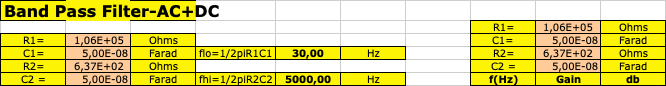Band Pass Filter-AC+DC
R1= 1,06E+05 Ohms
R1= 1,06E+05 Ohms C1= 5,00E-08 Farad
C1= 5,00E-08 Farad flo=1/2piR1C1 30,00 Hz R2= 6,37E+02 Ohms
R2= 6,37E+02 Ohms C2 = 5,00E-08 Farad
C2 = 5,00E-08 Farad fhi=1/2piR2C2 5000,00 Hz f(Hz) Gain db

Then I take the filter, plotted on a chart in the frequency domain (with values ​​between 0 and 1) and multiplied by my signal also entered in the frequency domain, so that it is filtered.

Code:

`````` QVector <double> x1, y_freq_sample;

double x2, y1;
x2 = y1 = 0;
for (int i=0;i<x_num;++i){
x2=plot8_0->at(i)->key;
y1=plot8_0->at(i)->value*plot8_1->at(i)->value; // plot8_0 (signal), plot8_1 (filter)
x.append(x2);
y_freq_sample.append(y1);
}
``````

Here my problem begin. I need a complex number to apply de FFTi. So I tried to adapt the recommendations you gave me and I did the following:

`````` QVector<kiss_fft_cpx> complexInput(frequencies.size()); // which is the same as frequencies

for (int i = 0; i < complexInput.size(); i++) {
complexInput[i].r = frequencies[i].r*plot8g1->at(i)->value; // my signal is frequencies[i].r and i need to multiply by the filter.
complexInput[i].i = frequencies[i].i;// I do not know what I should do here to adjust the phases
}

QVector<kiss_fft_scalar> samplingRecon(nfft);
bool inverserFFT = true; //FFTi
kiss_fftr_cfg icfg = kiss_fftr_alloc(nfft, inverserFFT, nullptr, nullptr);
kiss_fftri(icfg, complexInput.data(), samplingRecon.data());

QVector <double> x_time (nfft), y_time(nfft);
double time = (x_max2/x_num);

for(int i=0; i<nfft;i++){

x_time[i] = i*(time);
y_time[i] = samplingRecon.at(i);
}
free(icfg);
``````

//-------- Here i plot a x_time and y_time graph.

The graph looks almost normal (the frequencies are correct but there is a delay)

cheers

• Hi @pedromenezes,
In general you would apply a filter to your signal, right?

To understand what are you doing I have some questions:

double freqFFT = (1000/x_max2); //1000(msec)/time
...
x_freq[i] = i*freqFFT;

This is to convert the frequencies from `Hz` to `mHz`?

I also don't understand this part:

y_freq[i] = abs(frequencies[i].r)/180;

If you want to plot the amplitude, you should calculate it with:

``````    const double real = frequencies[i].r;
const double imag = frequencies[i].i;
y_freq[i] << qSqrt(real*real + imag*imag);
``````

To apply a filter to the signal, i'm not quite sure if you need to multiply your the filter value to the amplitude and not only to the real part (you can also maybe muliply the filter value with the real and imaginary part). The filter value is in general a function which depends on the frequency.

``````for (int i = 0; i < complexInput.size(); i++) {
const double amplitude = aplitudeSpectrum.at(i) * yourFilterValue(i);
const double phase = phaseSpectrum.at(i);

double real = amplitude * qCos(phase);
double imag = amplitude * qSin(phase);

complexInput[i].r = real;
complexInput[i].i = imag;
}
``````

You can also try this:

``````        for (int i = 0; i < complexInput.size(); i++) {
complexInput[i].r = frequencies[i].r * plot8g1->at(i)->value;
complexInput[i].i = frequencies[i].i * plot8g1->at(i)->value;
}
``````

• Thanks again @beecksche !

double freqFFT = (1000/x_max2); //1000(msec)/time
...
my x axis signal is expressed in msec. For that reason if a don't do this the frequency axis will be 1000 times greater.

y_freq[i] = abs(frequencies[i].r)/180; // to be more precise I change to y_freq[i] = abs(frequencies[i].r)/200.

I did this experimentally so that the spectrum amplitude in the frequency domain has the same value of the wave amplitude in the time domain. I have not really understood the reason why these amplitudes are different.

Before I went to sleep yesterday I still did some tests and I see today that once again you were right. It really worked when I multiplied the real part and also the imaginary part by the filter. The result went as I wanted. I still got a single doubt because two small distortions of the signal obtained with the FFTi (one at the beginning and one at the end) appear, I do not know if it is due to the low number of points (512), or another problem.

In the figure below, you can see the input wave with frequency of 1 kHz and amplitude of 1uV (blue), the signal after the FFT very close to 1kHz (green). The amount of points does not allow for better accuracy, but for what I need, it looks great. Finally, the FFTi (red), with exactly the same characteristics of the input wave.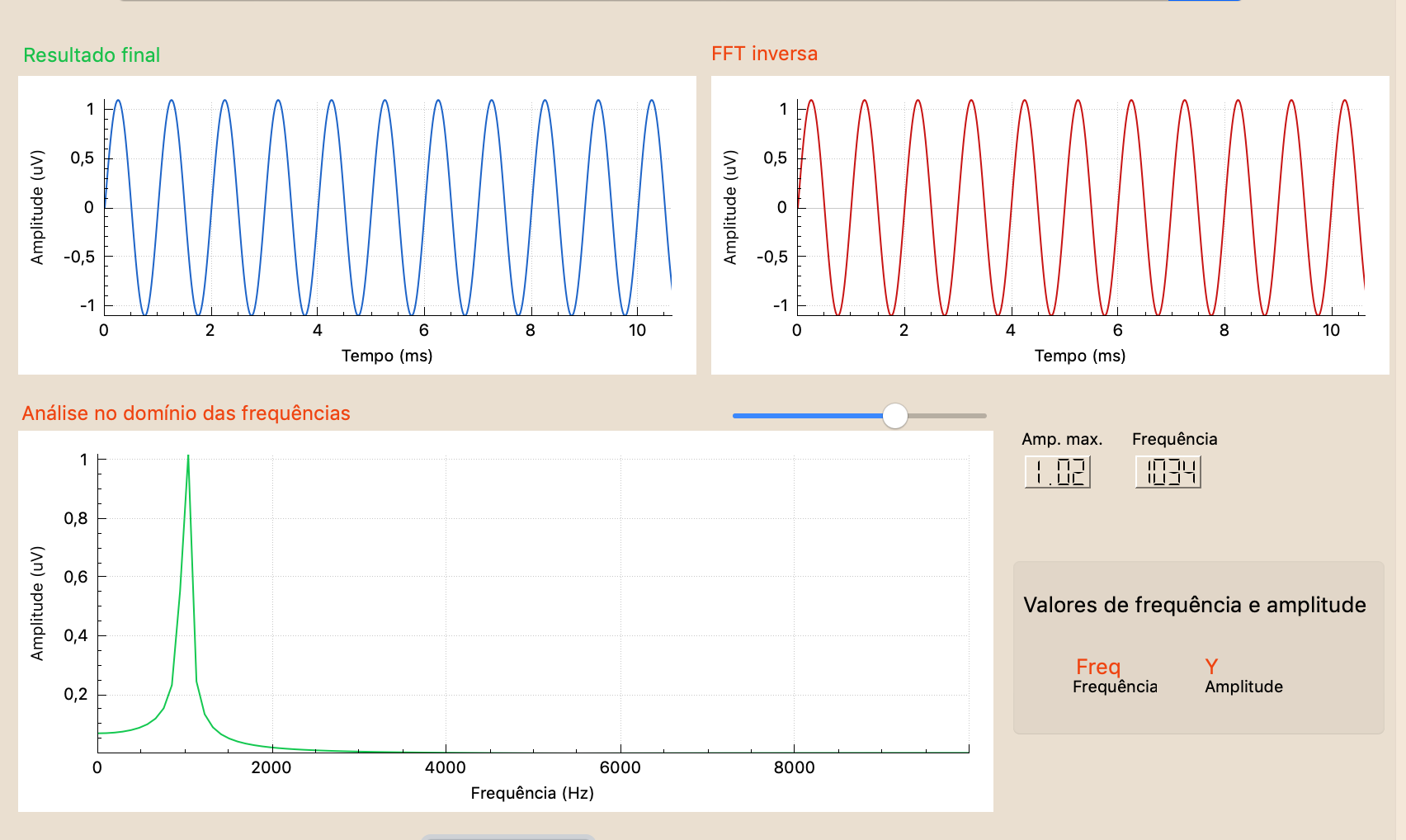In the following figure, you can see the input wave (blue) and the filtered wave (red). In this example I applied a filter that should not interfere with the signal (30 Hz - 5 kHz bandpass), since the signal had 1 kHz. But as you can see, there is a small phase and amplitude distortion at the beginning and at the end. For what I need, again it will not be a problem, yet it would be interesting to understand. Do you have any idea what it is?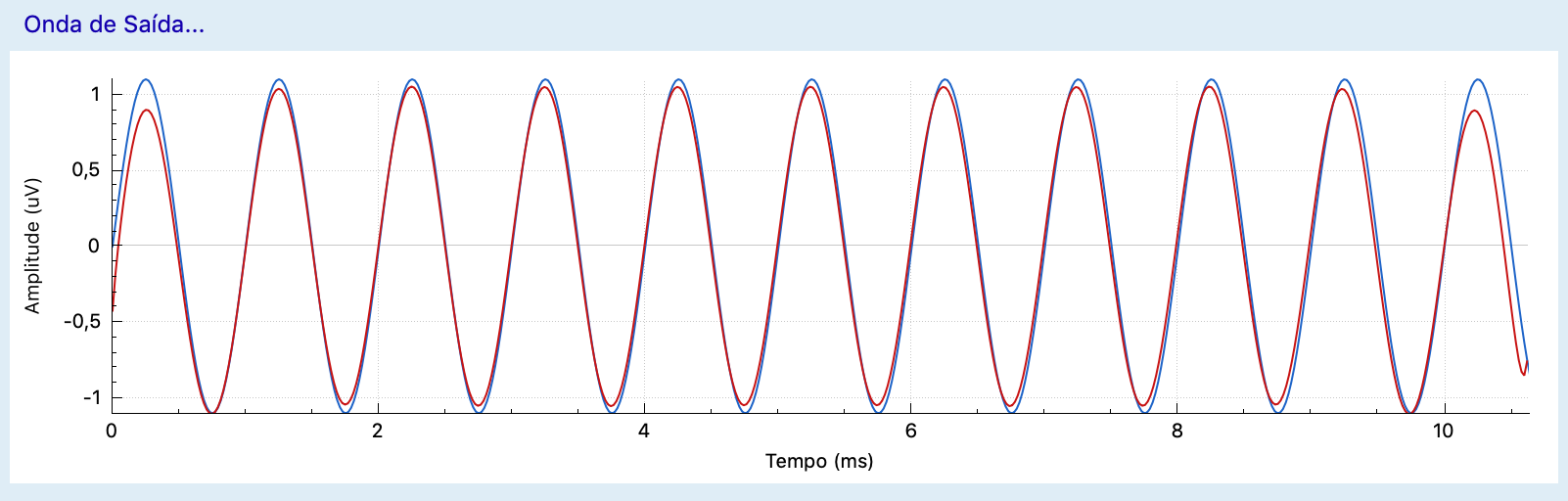Cheers

• double freqFFT = (1000/x_max2); //1000(msec)/time

I see, so `x_max2`are in msec.

The function

abs(frequencies[i].r)

is not the absolute (or modulus) value of a complex number. It is the standard math function std::abs?
Or do want to plot only the real part?

As said in my previous posts the modulus of a complex number need to be calculated with the real and imaginary part of the complex number. See Wikipedia:This amplitude (absolute value, or modulus of the complex number) need to be scaled. See also previous posts:

``````waveAmplitude = modulus * 2 / sampleRate
``````

First, the calculated modulus need to be multiplied with 2. Why?After the FFT of your sampled signal the spectrum is halfed, and only one side (positive) is calculated. The second (negative) side is the conjugate complex (just the opposite sign of the imaginary part). So the origin amplitude is halfed.

Second, the value need to be divided with the sample rate. Why? Many FFT do not normalize the complex values, because of performance reasons. See KissFFT and FFTW.

Why the filteres signal is distored? If you share the code, we may find something.

• Hi @beecksche,

I am convinced that the problem is in the code of my filter. I spent these days looking for a library ready to use and I found DspFilter. I was able to add it to my program, with some warnings (most of them is "warning: comparing floating point with == or != is unsafe"), and I'm still studying how to use it.

The filter that I currently have has the following code:

``````void promediation::Filter_analog_Plot(){

auto plot5 = prom->customPlot_5;
auto plot8= prom->customPlot_8;
int num = 512;

QVector <double> x (num), y_final(num);
double r1, c, r2, amos, pontos, pow1, eq1, eq2, pow2, eq3;
c = 0.00000005;
r1 = 1/(2*M_PI*prom->doubleSpinBox_4->value()*c);
r2 = 1/(2*M_PI*prom->doubleSpinBox_5->value()*c);
amos = prom->doubleSpinBox_3->value();
pontos = prom->spinBox->value();

double maior = 0;

for (int i=0;i<num;++i){

if (i==1){ // to correct a distortion on hi-pass filter
x = 0;
x = 1;
x = 5;
x = 95;
}

if (2<i&&i<12){ // to correct a distortion on hi-pass filter
x[i] = (i*10)-20;
}

if (i>12){
x[i]=(i-12)*amos/(pontos-1);
}

pow1 = pow(2*M_PI*r1*c*x[i],2)+1; // main function
eq1 = 1/sqrt(pow1);
eq2 = 2*M_PI*r2*c*x[i];
pow2 = pow(2*M_PI*r2*c*x[i],2)+1;
eq3 = sqrt(pow2);
y_final[i]= ((eq1*eq2)/eq3); // end of main function

if (y_final[i]> maior){
maior= y_final[i];
}

}
for (int n = 0; n < num; ++n){
y_final[n]=y_final[n]/maior;
}

plot5->graph(0)->setData((x), (y_final));
plot5->replot();
plot8->graph(1)->setData((x), (y_final));
plot8->replot();

}
``````

Filter with correction: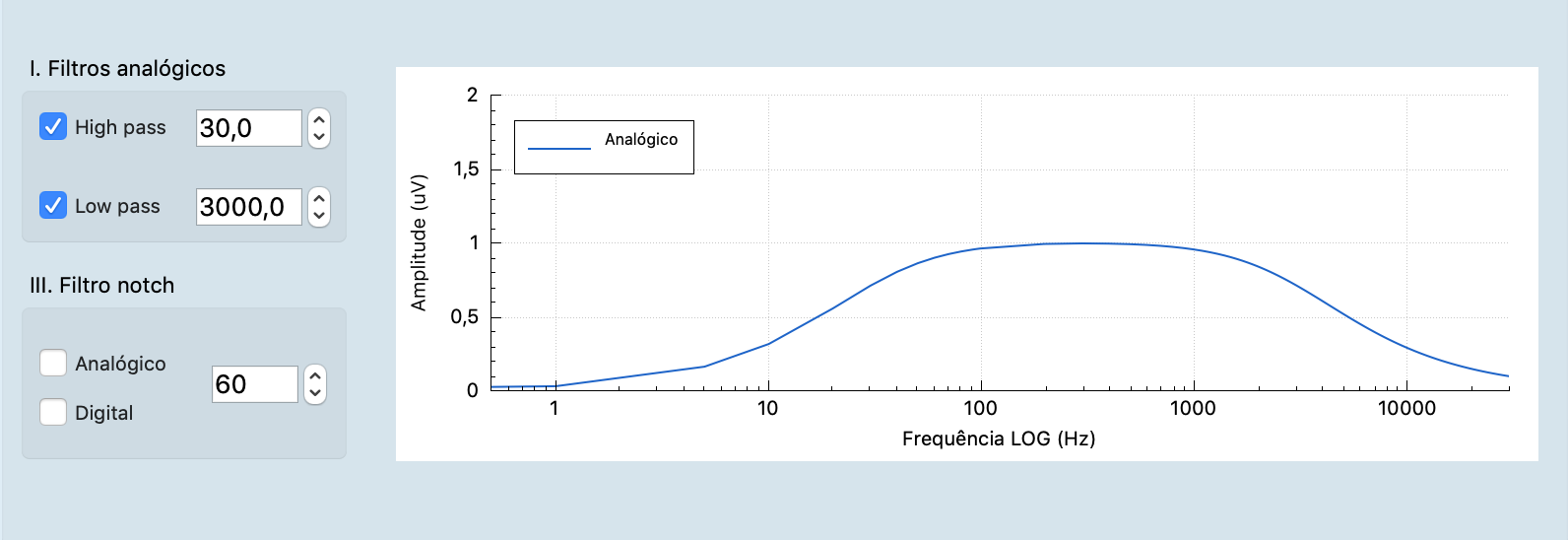Filter without correction code:

``````
x[i]=(i)*amos/(pontos-1);

pow1 = pow(2*M_PI*r1*c*x[i],2)+1; // main function
eq1 = 1/sqrt(pow1);
eq2 = 2*M_PI*r2*c*x[i];
pow2 = pow(2*M_PI*r2*c*x[i],2)+1;
eq3 = sqrt(pow2);
y_final[i]= ((eq1*eq2)/eq3);

if (y_final[i]> maior){
maior= y_final[i];
}

}
``````

Filter without correction :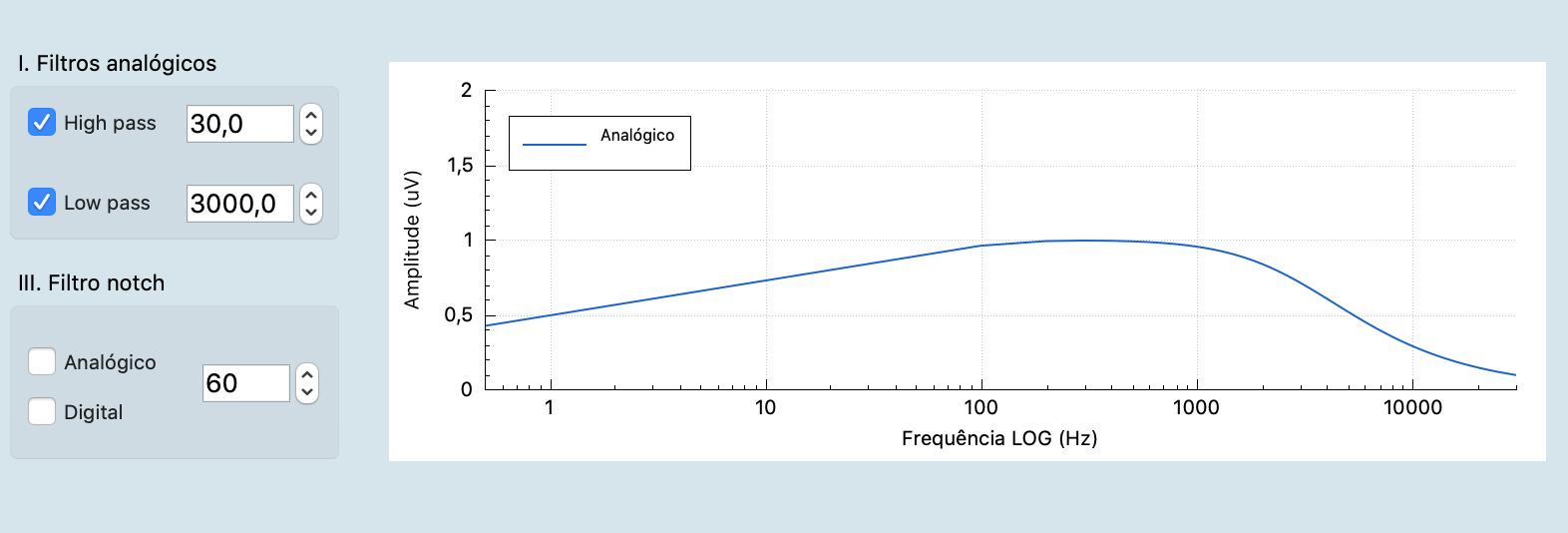``Cheers``